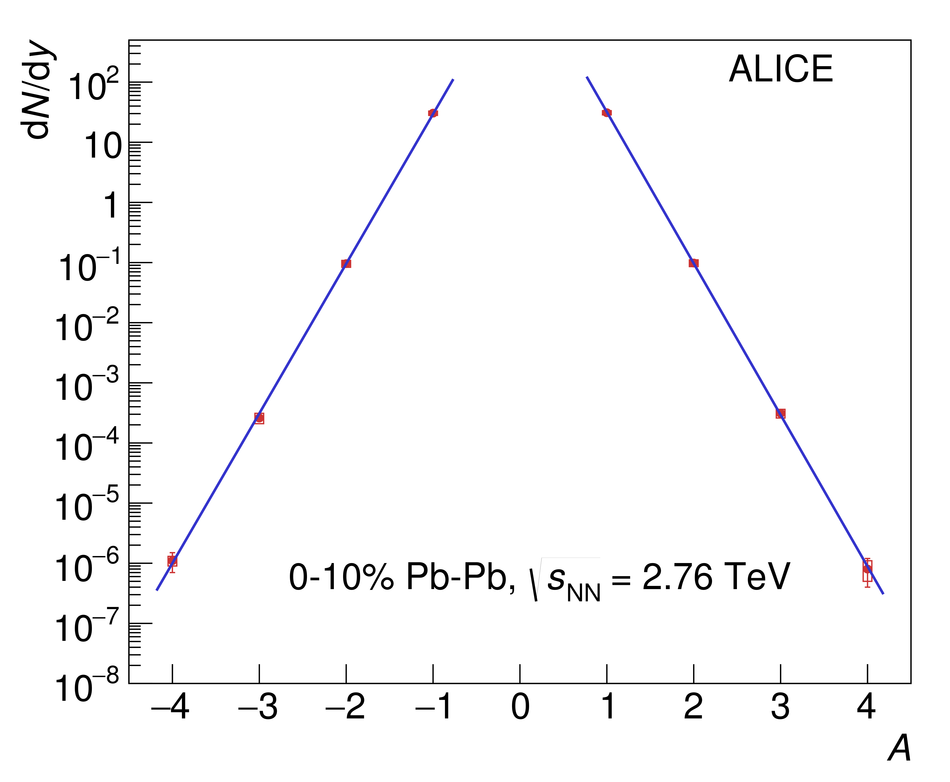# Production of $^{4}$He and $^{4}\overline{\textrm{He}}$ in Pb-Pb collisions at $\sqrt{s_{\mathrm{NN}}}$ = 2.76 TeV at the LHC

Results on the production of $^{4}{\textrm{He}}$ and $^{4}\overline{\textrm{He}}$ nuclei in Pb-Pb collisions at $\sqrt{s_{\mathrm{NN}}} = 2.76$ TeV in the rapidity range $\mid y \mid <~ 1$, using the ALICE detector, are presented in this paper. The rapidity densities corresponding to 0-10% central events are found to be $\mathrm{d}N/\mathrm{d}y _{^{4}\mathrm{He}} = (0.8 \pm 0.4 ~(\mathrm{stat}) \pm 0.3~(\mathrm{syst}))\times 10^{-6}$ and $\mathrm{d}N/\mathrm{d}y _{^{4}\mathrm{\overline{He}}} = (1.1 \pm 0.4~(\mathrm{stat}) \pm 0.2~(\mathrm{syst}))\times 10^{-6}$, respectively. This is in agreement with the statistical thermal model expectation assuming the same chemical freeze-out temperature ($T_{\mathrm{chem}}$ = 156 MeV) as for light hadrons. The measured ratio of $^{4}\overline{\mathrm{He}}$/$^{4}\mathrm{He}$ is $1.4 \pm 0.8~(\mathrm{stat}) \pm 0.5~(\mathrm{syst})$.

Figures

## Figure 1

 Velocity $\beta$ measured with the TOF detector as a function of the rigidity $p/z$. For this figure a selection band of -1.5 to 3$\sigma$ around the mean of the TPC specific energy-loss distribution is required Negatively (positively) charged particles are shown on the left (right) side, with positive tracks in blue and negative tracks in green. The dashed vertical line represents the cut on the rigidity $p/z = 2$~GeV/$c$ (applied only for positively charged particles). The insert shows the $m^2/z^2$ distributions obtained from the data points shown in the main figure.## Figure 2

 d$N$/d$y$ for protons (A=1) up to $^4$He (A=4) and the corresponding anti-particles in central (0-10\%) Pb--Pb collisions at $\sqrt{s_{\mathrm{NN}}}$ = 2.76 TeV. The blue lines are fits with an exponential function. Statistical uncertainties are shown as lines, whereas the systematic uncertainties are represented by boxes.## Figure 3

 Thermal model fits, with three different implementations, to the light flavour hadron yields in central (0-10\%) Pb--Pb collisions at $\sqrt{s_{\mathrm{NN}}}$ = 2.76 TeV. The data points are taken from~ and details of the fits can be found in~. The upper panel shows the fit results together with the data, whereas the middle panel shows the difference between model and data normalised to the model value and the lower panel the difference between model and data normalised to the experimental uncertainties.